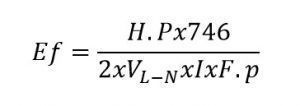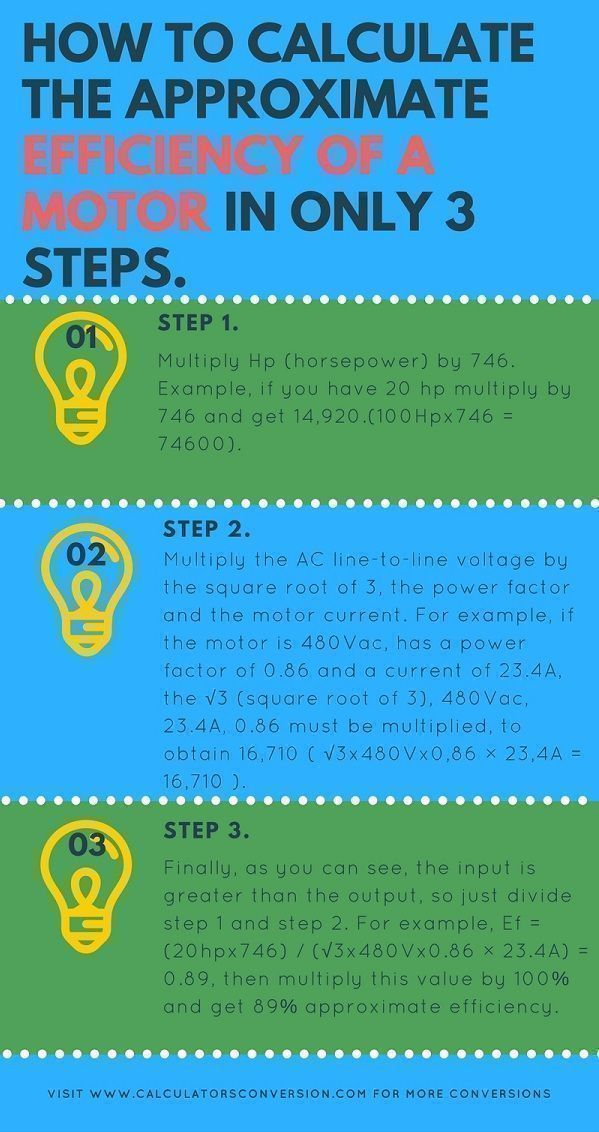# Automatic calculation of the efficiency of an electric motor, formula and table

With this efficiency calculator for motos you can convert and calculate online automatically in only 3 step, easy, fast and free any power associated with electric motors.

For greater ease we explain that formula uses the calculator with a table of the main efficiencies for motors, with different percentages of load.

We also show the most common power factors of different motors.

## Formula motor efficiency AC, biphasic:## Formula motor efficiency AC, three phase:##Step 1:

Multiply Hp (horsepower) by 746. Example, if you have 20 hp multiply by 746 and get 14,920.(100Hpx746 = 74600).

## Step 2:

Multiply the AC line-to-line voltage by the square root of 3, the power factor and the motor current. For example, if the motor is 480Vac, has a power factor of 0.86 and a current of 23.4A, the √3 (square root of 3), 480Vac, 23.4A, 0.86 must be multiplied, to obtain 16,710 ( √3x480Vx0,86 × 23,4A = 16,710 ).

## Step 3:

Finally, as you can see, the input is greater than the output, so just divide step 1 and step 2. For example, Ef = (20hpx746) / (√3x480Vx0.86 × 23.4A) = 0.89, then multiply this value by 100% and get 89% approximate efficiency.

## Definitions Efficiency, Hp, FP, Iac, Idc and Volts:

E (Efficiency) = The efficiency of the motor is the ratio between the amount of mechanical work performed and the electrical energy consumed to do the job, represented by a percentage. A higher percentage represents a more efficient engine. The efficiency of the electric motor depends on (but not limited to) the conditions of design, materials, construction, grading, loading, power quality, and operation.
The efficiency of any electric motor is its power output divided by the total input. To be valid, input and output must be expressed in the same physical units.

The input to an AC motor is the electric power in watts (or kilowatts), while the output of the motor is the mechanical energy delivered by the shaft, so the shaft torque by the speed (rpm). Starting from the fact that the output of the motor torque is not an electrical quantity, it is usually measured in mechanical horsepower units.

To divide that by the electric input into watts, the horsepower (hp) must be converted to the electrical equivalent by means of its equivalence (746 watt). (In the metric system, the watt or kilowatt is the measurement of both the output and the input, because different torque units are used.)

The input must always exceed the output, the difference being the internal energy loss in the motor. Thus, the output divided by the input can never equal or exceed the unit (100%).

FP = The power factor is the difference between the apparent power S (VA) and the actual or active power P (W) , this factor can be determined with the formula: P (W) / S (VA) = FP practice the power factor is determined by the characteristics of the equipment.

HP = The horsepower, also called power horse – since it is a measure of power and not of force – and in English horsepower, is the name of several units of measurement of power used in the Anglo-Saxon system. It is denominated hp, HP or Hp, of the English term horsepower, expression that was coined by James Watt in 1782 to compare the power of the steam engines with the power of the draft horses. It was later expanded to include the output power of other types of piston engines, as well as turbines, electric motors and other types of machinery.

Iac = The alternating current is described as the load flow that changes direction periodically, resulting in the voltage level also reversed in conjunction with the current. AC alternating current is used to power homes, office buildings, commerce, etc. This form of electrical energy is what consumers usually use when connecting kitchen appliances, televisions and electrical appliances to an electrical outlet.

Idc = The DC current refers to the continuous flow of electric charge through a conductor between two points of different potential, which does not change direction with time. Direct current is produced by sources such as batteries, power supplies, thermocouples, solar cells, or dynamos. Direct current can flow in a conductor such as a wire, but it can also flow through the semiconductors, insulators, or even through a vacuum such as in electron or ion beams. Direct current is used to charge the batteries and as a power source for electronic systems.

Volts = The volt is the unit of the electric potential difference (voltage). The Volt is named in honor of the Italian physicist Alessandro Volta (1745-1827), who invented the voltaic battery, possibly the first chemical battery.

## Typical Motor Power Factors:

 Power Speed Power Factor (hp) (rpm) 1/2 load 3/4 load full load 0 – 5 1800 0.72 0.82 0.84 5 – 20 1800 0.74 0.84 0.86 20 – 100 1800 0.79 0.86 0.89 100 – 300 1800 0.81 0.88 0.91

Reference // Power Factor in Electrical Energy Management-A. Bhatia, B.E.-2012
Power Factor Requirements for Electronic Loads in California- Brian Fortenbery,2014
http://www.engineeringtoolbox.com

## NEMA Design B Electrical Motors Efficiency

Electrical motors constructed according NEMA Design B must meet the efficiencies below:

Power
(hp)
Minimum Nominal Efficiency1)
1 – 4 78.8
5 – 9 84.0
10 – 19 85.5
20 – 49 88.5
50 – 99 90.2
100 – 124 91.7
> 125 92.4

1) NEMA Design B, Single Speed 1200, 1800, 3600 RPM. Open Drip Proof (ODP) or Totally Enclosed Fan Cooled (TEFC) motors 1 hp and larger that operate more than 500 hours per year.

Qualify efficiency calculator for electric motor:  [kkstarratings]